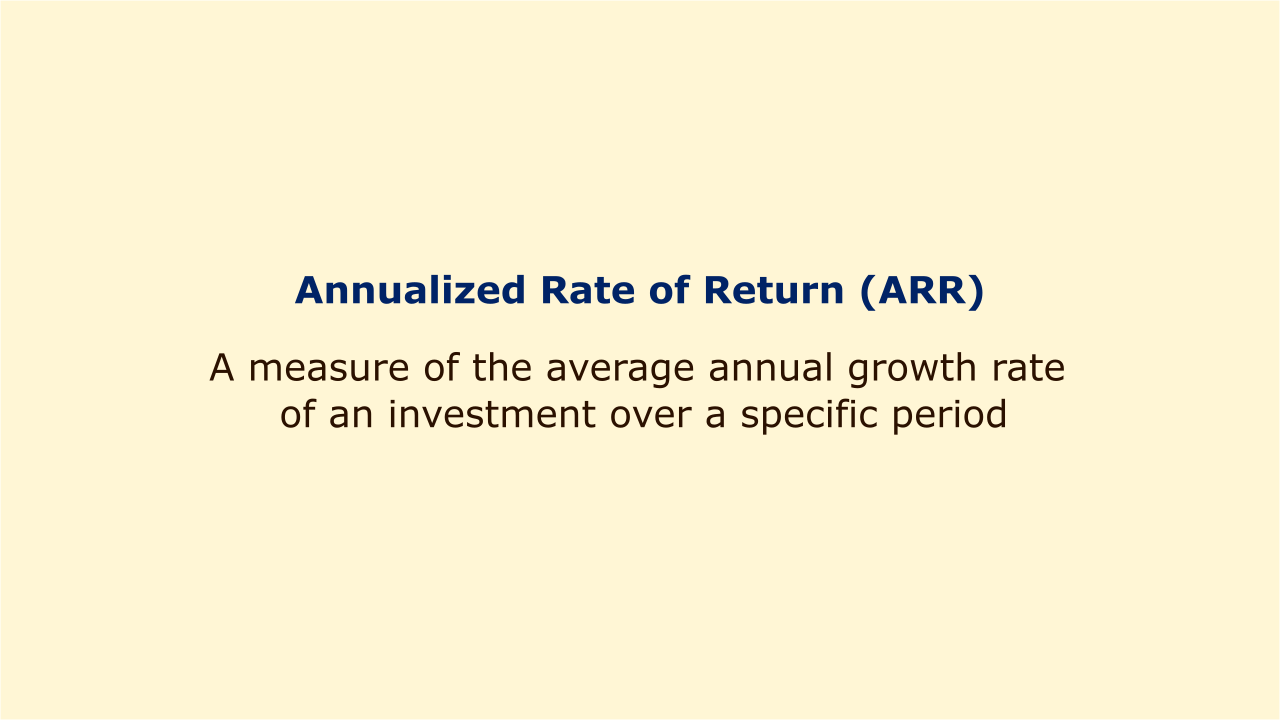# Annualized Rate of Return (ARR)Image: Moneybestpal.com

### Annualized Rate of Return (ARR) is a measure of the average annual growth rate of an investment over a specific period. It can be used to assess portfolio growth or to compare the performance of various investments.

ARR considers the compounding effect of investment returns over time, therefore it accounts for interest accrued on both the principal and the cumulative interest.

We need to know the initial investment's value, the final investment's value, and how many years the first investment was held in order to compute ARR. The formula for ARR is:

ARR = ((Ending Value / Beginning Value) ^ (1 / Number of Years)) - 1

The outcome is multiplied by 100 to produce the result as a percentage. ARR indicates if an investment has increased in value over time. A negative ARR indicates whether an investment has decreased in value over time.

Assume, for instance, that an investor put \$10,000 into a mutual fund, and five years later the investment was worth \$15,000. The ARR of this investment is:

ARR = ((\$15,000 / \$10,000) ^ (1 / 5)) - 1

ARR = (1.5 ^ 0.2) - 1

ARR = 0.0845 - 1

ARR = 0.0845

Multiplying by 100, we get:

ARR = 8.45%

This means that the investment grew by an average of 8.45% per year over the five-year period.

ARR, however, says nothing about the actual yearly returns during the time period, which may differ greatly from year to year. For instance, the same investment might have yearly returns of 10%, 5%, 15%, -2%, and 12% over the course of five years, but the final investment would still be worth \$15,000 and have an ARR of 8.45%. As a result, ARR should be utilized with care and combined with other risk and return indicators, such as standard deviation, the Sharpe ratio, or alpha.
Tags